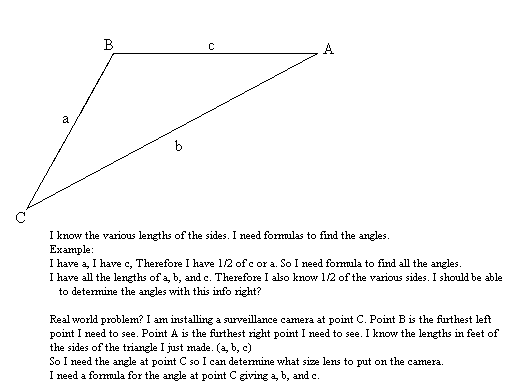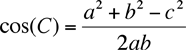How do you find the angles in a triangle if you know the lengths of the sides? Hi Keith, I reproduced what you sent us.There is an expression in trigonometry called the Law of Cosines that will give you what you need. Using your notation the Law of Cosines states c2 = a2 + b2 - 2 a b cos(C) ThusUse this expression to find cos(C) and then use the inverse cosine function (the cos-1 button on your calculator) to find C. Chris and Penny